Next: Output file content Up: IAC-star input/output guide Previous: IAC-star input/output guide

# IAC-star input parameters

The input parameters that the user should supply to IAC-star are the following:

• A seed for the random number generator. If 0 is entered, the computer will automatically generate it from its internal clock. The routine used for random number generation is ran2'', obtained from Numerical Recipes in Fortran (Press et al. 1997).

• Total number of stars computed or saved into the output file. The program will stop when the first of these numbers is reached. To prevent too long runs, the maximum allowed total computed star number is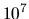stars and the maximum allowed number of saved stars is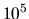. These limits may change in future versions of the program.

• Logarithm of the minimum stellar luminosity or maximum magnitude in a given filter. Although fainter stars are computed, only those brighter than this value are saved into the output file. In the case of binary stars, this value is applied to total luminosities or magnitudes.

• The SFR,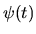, sampled by a number of points (up to 20 in the current version). The number of points, including first and last, is given first. For a SFR defined by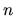points, the input is a list of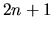numbers of the form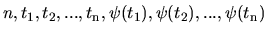, where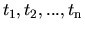are time instants and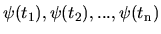, the corresponding SFR values. The age of the system,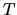, is asumed to be the last instant of time given,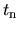. For an arbitrary instant of time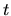, the SFR,is computed by simple linear interpolation between the two points embracing it; i.e. between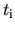and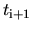, such that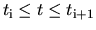. The time stepsmust be given in Gyr. Units for the SFR,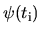, are arbitrary. In fact, they are defined upon the end of the execution by the integral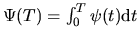, stored in the output file.

• The chemical enrichment law. Two alternative approaches are allowed to produce the chemical enrichment law: (i) just an interpolation in several age-metallicity nodes, defined by the user and permiting a completely arbitrary metallicity law; (ii) computation from usual parameters involved in physical scenarios of chemical evolution, even though a self-consistent physical formulation is not intended in this case. We describe separetely the input parameters of each approach in the following.

• (i) In the interpolated CEL case, the values ofand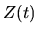for the nodes are given in a similar way as for the SFR above. This includes a simple, lineal CEL, varying from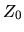,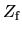and the obvious case of constant metallicity (for which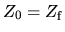).

It is usefull for many purposes to allow metallicity dispersion at any time. For this, a second metallicity law can be introduced. If so, for any time, actual metallicities of synthetic stars are randomly distributed between the values of the two laws. If an unique, well defined metallicity for each age is required, the parameters of the second law must be all them 0 or leaved blank.

• (ii) The following ecuations are used to compute the chemical law for infall and outflow: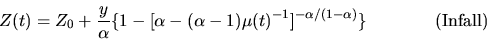(1)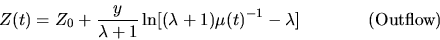(2)andare the initial and final metallicities;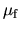is the final gas fraction, and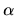and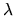are the infall and outflow parameters. These parameters are provided in the program input. Constant yield and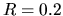are assumed. The latter is obtained for a stellar system in which star formation has gone on at a constant rate from 13 Gyr ago to date. Small, probably not significant differences are found for other SFRs and depending on metallicity.

Since they are governed by different equations, infall and outflow can not be included simultaneously. However, an approximation is obtained if the infall case is used with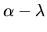as parameter. It must be noted, however, that the approximation refers only to the CEL morphology, but not to the physical scenario. In particular, the yield becomes rather unrealistic.

In general, the infall and outflow parameters should be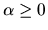and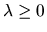to have physical sense. Moreover they must verify the conditions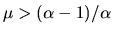, for the infall scenario, and, for the outflow one. The cases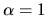,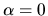(infall scenario) and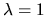(outflow scenario), are overcome by the code by substituting these values by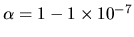;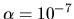, and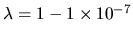respectively. In this way, the code can produce the closed-box case and the infall limit case, in which infall is balanced by star formation.

As in the case (i), a second law can be defined if metallicity dispersion is required.

It must finally be mentioned that, in any case, the metallicity distribution is truncated to the range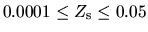, which are the current limits of the used library.

• The IMF. It is assumed to be a power law of the mass, but several mass intervals can be defined. The user can set here the minimum and maximum stellar masses to be computed. It must be noted that, in most cases, the execution time can be considerably reduced by rising the minimum stellar mass.

• Binary star control. Both the fraction of binary stars and the secondary to primary minimum mass ratio can be supplied. If the first is 0, no binaries are computed. About the second, only the minimum value is requested, the maximum one being 1.

• Mass loss parameter. Although the default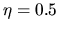seems a reasonable choice, the user can modify it here. It must be noted that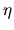significantly affects the extension of the HB for low metallicity stars.Next: Output file content Up: IAC-star input/output guide Previous: IAC-star input/output guide
Antonio Aparicio 2003-10-29